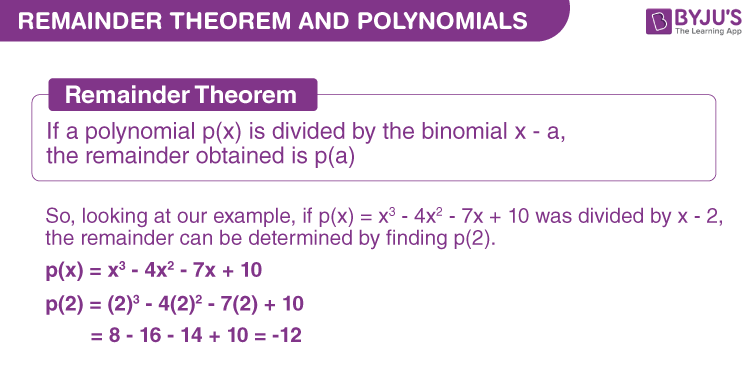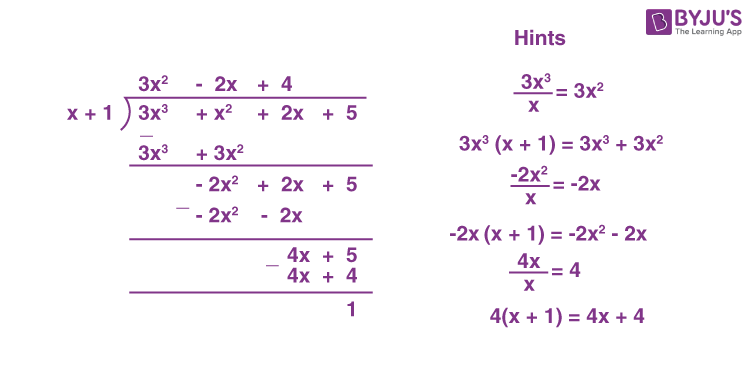# Remainder Theorem

Remainder Theorem is an approach of Euclidean division of polynomials. According to this theorem, if we divide a polynomial P(x) by a factor ( x – a); that isn’t essentially an element of the polynomial; you will find a smaller polynomial along with a remainder. This remainder that has been obtained is actually a value of P(x) at x = a, specifically P(a). So basically, x -a is the divisor of P(x) if and only if P(a) = 0. It is applied to factorize polynomials of each degree in an elegant manner.

For example: if f(a) = a3-12a2-42 is divided by (a-3) then the quotient will be a2-9a-27 and the remainder is -123.

if we put, a-3 = 0

then a = 3

Hence, f(a) = f(3) = -123

Thus, it satisfies the remainder theorem.## Remainder Theorem Definition

The Remainder Theorem begins with a polynomial say p(x), where “p(x)” is some polynomial p whose variable is x. Then as per theorem, dividing that polynomial p(x) by some linear factor x – a, where a is just some number. Here go through a long polynomial division,  which results in some polynomial q(x) (the variable “q” stands for “the quotient polynomial”) and a polynomial remainder is r(x). It can be expressed as:

p(x)/x-a = q(x) + r(x)

Factor Theorem

Factor Theorem is generally applied to factoring and finding the roots of polynomial equations. It is the reverse form of the remainder theorem. Problems are solved based on the application of synthetic division and then to check for a zero remainder.

When p(x) = 0 then y-x is a factor of the polynomial Or if we consider the other way, then When y-x is a factor of the polynomial then p(x) =0

### Remainder Theorem Proof

Theorem functions on an actual case that a polynomial is comprehensively dividable, at least one time by its factor in order to get a smaller polynomial and ‘a’ remainder of zero. This acts as one of the simplest ways to determine whether the value ‘a’ is a root of the polynomial P(x).

That is when we divide p(x) by x-a we obtain

p(x) = (x-a)·q(x) + r(x),

as we know that Dividend = (Divisor × Quotient) + Remainder

But if r(x) is simply the constant r (remember when we divide by (x-a) the remainder is a constant)…. so we obtain the following solution, i.e

p(x) = (x-a)·q(x) + r

Observe what happens when we have x equal to a:

p(a) = (a-a)·q(a) + r

p(a) = (0)·q(a) + r

p(a) = r

Hence, proved.

### Steps to Divide a Polynomial by a Non-Zero Polynomial

• First, arrange the polynomials (dividend and divisor) in the decreasing order of its degree
• Divide the first term of the dividend by the first term of the divisor to produce the first term of the quotient
• Multiply the divisor by the first term of the quotient and subtract this product from the dividend, to get the remainder.
• This remainder is the dividend now and divisor will remain same
• Again repeat from the first step, until the degree of the new dividend is less than the degree of the divisor.

## Remainder Theorem of Polynomial

Let us understand the remainder theorem in polynomials with the example given below:

Divide 3x3 + x2 + 2x + 5 by x + 1.

Solution:

From the given,

Dividend = p(x) = 3x3 + x2 + 2x + 5

Divisor = g(x) = (x + 1)Here, quotient = q(x) = 3x2 – 2x + 4

Remainder = r(x) = 1

Verification:

Given, the divisor is (x + 1), i.e. it is a factor of the given polynomial p(x).

Let x + 1 = 0

x  = -1

Substituting x = -1 in p(x),

p(-1) = 3(-1)3 + (-1)2 + 2(-1) + 5

= 3(-1) + 1 – 2 + 5

= -3 + 4

= 1

Remainder  = Value of p(x) at x = -1.

Hence proved the remainder theorem.

Alternatively,

p(x) = (x – a)·q(x) + r

Observe what happens when we have x equal to a:

p(a) = (a – a)·q(a) + r

Substituting the values,

p(-1) = [-1 – (-1)]·q(-1) + (-1)

p(-1) = 0.q(-1) – 1

p(-1) = -1

p(-1) = remainder

Hence proved.

### Remainder Theorem Problems

Consider the following example:-

Example- Determine that x = 1 is a root of P(x),

Explanation:

It suggests that x = 1 may be a root of P(x), and (x – 1) may be a factor of P(x)

Then if we tend to divide synthetically from P(x) by (x – 1), we will get a new smaller polynomial and a remainder of zero:

 Example: Find the root of the polynomial x2 – 3x – 4. Solution: $$\begin{array}{l} x^{2} – 3x – 4\end{array}$$ $$\begin{array}{l} f(4) = 4^{2} – 3(4) – 4\end{array}$$ $$\begin{array}{l} f(4) = 16 – 16 = 0\end{array}$$ So,  (x-4) must be a factor of x2 – 3x – 4. Example: Find the remainder when t3 -2t2 + t + 1 is divided by t – 1. Solution: Here, p(t) = t3 -2t2 + t + 1, and the zero of t – 1 is 1. ∴ p (1) = (1)3 – 2(1)2 + 1 + 1= 2 By the Remainder Theorem, 2 is the remainder when t3 -2t2 + t + 1 is divided by t – 1.

### Euler Remainder Theorem

Euler’s theorem  states that if n and X are two co-prime positive integers, then
Xφ(n) = 1 (mod n)

where, φ(n) is Euler’s function or Euler’s totient function, which is equal to;

φ(n) = n (1-1/a).(1-1/b).(1-1/c)

where, n is a natural number, such that n = ap. bq . cr,

Here, a, b, c are prime factors of n and p, q, r are positive integers.

 Example: Find the Euler totient function of 35. Solution: The factors of 35 are as follows: $$\begin{array}{l}35 = 5 \times 7\end{array}$$ $$\begin{array}{l} φ(35) = 35 \left ( 1 – \frac{1}{5} \right ) . \left ( 1 – \frac{1}{7} \right ) = 24 \end{array}$$ Thus the totient function of 35 is 24.
$$\begin{array}{l}Questions\ of\ the\ form: \ \mathbf{\frac{m^{a}}{n}}\end{array}$$
 $$\begin{array}{l}Example: Find\ the\ remainder\ when\ 3^{76} \ is\ divided\ by\ 35.\end{array}$$ Solution: Here m = 3, a = 76 and n = 35, In an example above, we have already found the totient function of 35, which is equal to 24. $$\begin{array}{l}Remainder\ of\ \frac{76}{\varphi (35)} = \frac{76}{24}= 4\end{array}$$ Remaining power is 4, which when divided by 35 given the resultant remainder. $$\begin{array}{l}which\ is,\ \frac{3^{4}}{35} = \frac{81}{35} = 11\end{array}$$ Thus the remainder comes out to be 11.

Test your knowledge on Remainder Theorem